# JP5600627B2 - Transmitter, receiver, transmission method and reception method - Google Patents

## Info

Publication number
JP5600627B2
JP5600627B2 JP2011070924A JP2011070924A JP5600627B2 JP 5600627 B2 JP5600627 B2 JP 5600627B2 JP 2011070924 A JP2011070924 A JP 2011070924A JP 2011070924 A JP2011070924 A JP 2011070924A JP 5600627 B2 JP5600627 B2 JP 5600627B2
Authority
JP
Japan
Prior art keywords
signal sequence
shift amount
unit
sequence
frequency
Prior art date
Legal status (The legal status is an assumption and is not a legal conclusion. Google has not performed a legal analysis and makes no representation as to the accuracy of the status listed.)
Active
Application number
JP2011070924A
Other languages
Japanese (ja)
Other versions
JP2012205282A (en
Inventor

Original Assignee

Priority date (The priority date is an assumption and is not a legal conclusion. Google has not performed a legal analysis and makes no representation as to the accuracy of the date listed.)
Filing date
Publication date
Application filed by 株式会社Ｎｔｔドコモ filed Critical 株式会社Ｎｔｔドコモ
Priority to JP2011070924A priority Critical patent/JP5600627B2/en
Publication of JP2012205282A publication Critical patent/JP2012205282A/en
Application granted granted Critical
Publication of JP5600627B2 publication Critical patent/JP5600627B2/en
Application status is Active legal-status Critical
Anticipated expiration legal-status Critical

## Images

•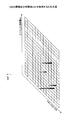•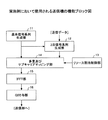•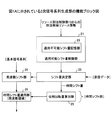••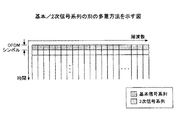•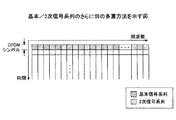•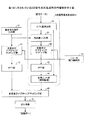••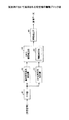•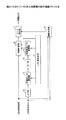•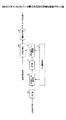•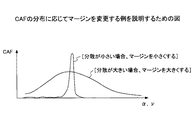•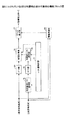•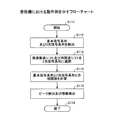•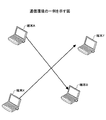•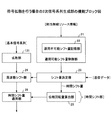•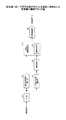•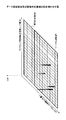••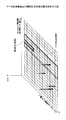## Description

The present invention relates to a transmitter, a receiver, a transmission method, and a reception method in a mobile communication system.

2. Description of the Related Art Conventionally, there is a technique that enables a receiver to detect the presence or absence of a specific system by adding a waveform feature amount to a signal transmitted by a transmitter. The waveform feature amount is an amount representing the statistical properties of a radio signal, and for example, a second-order cyclic autocorrelation function (CAF) can be used. This type of technology is described in Patent Document 1, for example. Furthermore, when generating a transmission signal by performing IFFT, there is a technique of applying a frequency shift or a time shift to a signal sequence and using the shift amount as a waveform feature amount.

JP 2008-61214

However, the conventional method has the following problems, for example.

1． When the frequency shift amount is used as the waveform feature amount, the frequency shift amount is determined by the position of the radio resource of the basic signal sequence to be transmitted (for example, the reference signal) and the radio resource of the secondary signal sequence derived from the basic signal sequence. And the amount of phase rotation added in symbol units such as OFDM symbols. In this case, a plurality of OFDM symbols to which various phase rotation amounts are added are necessary, and there is a problem that a plurality of OFDM symbols are necessary to realize one frequency shift amount.

2. When the presence / absence of a signal is detected using the second-order periodic autocorrelation value CAF, the presence / absence of the signal is determined based on the magnitude of the correlation value. When calculating the correlation, interference occurs between the signal to be detected and another signal, so that there is a problem that the detection accuracy of the presence / absence of the signal is deteriorated by the presence of the signal that is not the detection target.

3. When a single basic signal sequence or secondary signal sequence is transmitted on some subcarriers in the entire band, the amount of time shift that can be applied is limited. There is a problem that an inappropriate time shift amount is used.

Four. When a plurality of users use the same frequency at the same time, there is a problem that if a signal is identified only by time shift or frequency shift, the number of distinguishable users cannot be made sufficiently large.

Five. The same peak when the basic signal sequence length is short, when a certain time shift or frequency shift amount is added to a basic signal sequence, and when another time shift or frequency shift is added to the basic signal sequence Occurs and it is not easy to identify them.

6. When determining the presence or absence of the peak of the second-order periodic autocorrelation value, the conventional technique has a problem that the calculation burden is large.

The problem of the present invention is to solve one or more of the above problems.

The transmitter in one embodiment is:
A basic signal sequence generator for generating a basic signal sequence;
A secondary signal sequence that generates a secondary signal sequence that is cyclically shifted in the frequency domain by the specified frequency shift amount and cyclically shifted in the time domain by the specified time shift amount. A generator,
Multiplexer for multiplexing the basic signal sequence and the two signal sequence,
IFFT unit that performs inverse Fourier transform on the output from the multiplexing unit;
And a transmitter that transmits a transmission signal including an output from the IFFT unit.

According to one embodiment, one or more of the above problems can be solved.

The figure for demonstrating the 2nd period autocorrelation value CAF. The functional block diagram of the transmitter used in an Example. 1B is a functional block diagram of a secondary signal sequence generation unit shown in FIG. 1A. FIG. The figure which shows the multiplexing method of a basic / secondary signal sequence. The figure which shows another multiplexing method of a basic / secondary signal sequence. The figure which shows another multiplexing method of a basic / secondary signal sequence. The figure which shows the alternative example of the secondary signal sequence production | generation part shown by FIG. 1B. The flowchart which shows the operation example in a transmitter. The functional block diagram of the receiver used in an Example. FIG. 7 is a functional block diagram of the two-dimensional correlation detection unit shown in FIG. FIG. 8 is a detailed functional block diagram of the threshold value determination unit shown in FIGS. 6 and 7; The figure for demonstrating the example which changes a margin according to distribution of CAF. FIG. 7 is a functional block diagram of an alternative example of the two-dimensional correlation detection unit shown in FIG. The flowchart which shows the operation example in a receiver. The figure which shows an example of a communication environment. The functional block diagram of the secondary signal sequence production | generation part in the case of performing code spreading. The functional block diagram of the receiver used when code spreading is performed in the transmitter. The figure which shows the example of a setting of a data transmission area | region and a threshold determination area | region. The figure which shows the example of a setting of a data transmission area | region and a threshold determination area | region. The figure which shows the example of a setting of a data transmission area | region and a threshold determination area | region.

Regarding the first problem, by performing cyclic shift in the frequency domain on the signal to be transmitted, even if there is only one OFDM symbol, a signal shifted in frequency by a desired amount can be created. Become. However, in order to realize this, since it is necessary to accurately detect the position of the symbol where the basic signal sequence and the secondary signal sequence are arranged, arrangement information of these sequences and frequency synchronization information are required. . The arrangement information includes, for example, information indicating to which frequency subcarrier each sequence is mapped. The frequency synchronization information includes, for example, information such as the signal center frequency, bandwidth, and symbol timing.

Regarding the second problem, the base signal sequence and the secondary signal sequence are respectively extracted from the received signal by assuming that the position of the radio resource through which the basic signal sequence and the secondary signal sequence are transmitted is known on the receiver side. It becomes possible. For this reason, when calculating the correlation about the signal to be detected, it is possible to easily prevent the possibility of interference due to the signal that is not the detection target.

Regarding the third problem, as described later, it is possible to know an applicable time or frequency shift amount based on applied radio resource information or the like.

Regarding the fourth problem, when generating a secondary signal sequence, by multiplying the basic signal sequence by code spreading and appropriately using the spreading code to multiply, it is possible to distinguish user signals using the same frequency at the same time To do.

Regarding the fifth problem, it is possible to prevent deterioration in transmission quality by verifying in advance a pair of a highly correlated sequence and a shift amount and not using these shift amounts.

Regarding the sixth problem, by using a sequence with a length equal to or equivalent to the number of points of Fast Fourier Transform FFT or Inverse Fast Fourier Transform IFFT, and using a common threshold for multiple peak positions, the computational complexity Can be reduced.

Examples will be described from the following viewpoints.

1． Waveform features
2． Transmitter
3． Receiving machine
Four. First modification
Five. Second modification

<1. Waveform features>
The signal waveform is determined by various communication parameters such as the center frequency, frequency bandwidth, transmission power, modulation method, transmission symbol, etc. Conversely, the signal waveform is characterized by the above communication parameters. It is included. Such a feature is referred to as a “waveform feature” or “feature” in the present application. The waveform feature amount is information regarding statistical characteristics of the signal waveform and can be expressed in various amounts. For example, the waveform feature amount is expressed by periodic continuity obtained by a secondary periodic autocorrelation value, a signal amplitude variance value, a frequency correlation value, or the like.

In an embodiment described below, a predetermined waveform feature amount is given to a signal transmitted by a transmitter by applying a frequency shift and a time shift. The receiver can detect the presence / absence of a specific signal by analyzing the received signal and detecting such a waveform feature amount. Although the waveform feature amount can be expressed in various amounts, in the following description, a cyclic autocorrelation function (CAF) of a signal is used as the waveform feature amount. In this case, due to the nature of the signal (for example, the data modulation scheme, etc.), the periodic self only when certain unique parameters (cyclic frequency α and lag parameter ν) are used in the calculation of the periodic autocorrelation value. The property that the correlation value CAF increases is used. Also, different periodic steadiness feature quantities can be given to signals using the same modulation method.

In general, the value (CAF) of the second-order periodic autocorrelation function for the signal x (t) is calculated by the following equation.

Here, * represents a complex conjugate. I represents the observation time length. α represents a cyclic frequency or a frequency shift amount described later. τ represents a lag parameter or a time shift amount described later.

Regarding the second-order periodic autocorrelation value CAF, generally, if R x α (τ) ≠ 0 when α ≠ 0, x (t) has periodic stationarity.

Also, the discrete time expression of equation (1) is as follows.

Here, I 0 represents the number of observation samples. ν represents a discrete time representation of the lag parameter. Note that x [i] ≡x (iTs), and Ts represents a sampling period.

As shown in FIG. 1A, the second-order periodic autocorrelation value CAF takes a large value (peak value) for a specific parameter combination (α, ν), and a small value for other parameter combinations. Take. In this embodiment, the signal to be transmitted is cyclically shifted or cyclically shifted by a certain amount in the frequency axis direction, thereby shifting the position where the peak of the signal appears in the frequency axis direction. Further, the signal to be transmitted is cyclically shifted or cyclically shifted by a certain amount in the time axis direction, thereby shifting the position where the peak of the signal stands in the time axis direction. For example, when the peak of the second-order periodic autocorrelation value CAF of a signal occurs at (α, ν), the peak is generated by cyclically shifting the signal in the frequency axis direction and cyclically shifting in the time axis direction. The position can be changed to (α + Δα, ν + Δν). Therefore, for example, if the combination (α, ν) of the cyclic shift amount at the position where the peak occurs and specific data are associated in advance, the receiver determines the peak position, so that the transmitted data is determined. You can determine what it is.

<2. Transmitter>
FIG. 1B shows a functional block diagram of a transmitter used in this embodiment. FIG. 1B shows functional units or processing units particularly related to the present embodiment among various functional elements provided in the transmitter. The transmitter includes a basic signal sequence generation unit 11, a secondary signal sequence generation unit 12, a resource allocation control unit 13, a multiplexing and subcarrier mapping unit 14, a fast inverse Fourier transform unit (IFFT) 15, and a guard interval (GI) adding unit At least 16 are provided.

The basic signal sequence generation unit 11 generates a signal sequence by any method known in the art. The signal sequence can be any signal transmitted by the transmitter, but is a signal sequence that serves as a basis for generating a secondary signal sequence to be described later. The basic signal sequence generation unit 11 generates a basic signal sequence by applying error correction coding, interleaving, symbol mapping, and the like to the data signal sequence transmitted by the user. Here, when any method known in the art is used, for example, an M series, a gold series, or a part of these series may be mapped to a symbol such as BPSK. The sequence length may be adjusted by adding a null symbol or the like to the symbol sequence after being mapped to the symbol. Here, the sequence length of the signal is preferably 1 / 2n of the number of FFT points (n is a natural number).

The secondary signal sequence generation unit 12 generates a secondary signal sequence based on the basic signal sequence. The secondary signal sequence generation unit 12 will be described later with reference to FIG.

The resource allocation control unit 13 determines the position of the radio resource used to transmit the basic signal sequence and the secondary signal sequence. For example, when the transmitter is a base station, radio resources may be determined by scheduling based on radio channel conditions. If the transmitter is a user apparatus, the resource allocation control unit 13 may output a signal indicating what radio resource is designated by the base station. l
The multiplexing and subcarrier mapping unit 14 multiplexes or maps the basic signal sequence and the secondary signal sequence to the resource specified by the resource allocation control unit 13 in the time axis direction and the frequency axis direction. There are various ways of multiplexing or mapping.

FIG. 3A shows an example (frame structure) of a method of multiplexing the basic signal sequence and the secondary signal sequence by the TDM method. For convenience of explanation, it is assumed that a frame includes a plurality of orthogonal frequency division division (OFDM) symbols (OFDM symbols). In the case of the illustrated example, the OFDM symbol of the secondary signal sequence is transmitted after the OFDM symbol of the basic signal sequence using all subcarriers over the entire system band. It is not essential that the subcarriers used are the entire system band, and the basic signal sequence and the secondary signal sequence may be transmitted using some of the subcarriers.

FIG. 3B shows another method example (frame structure) for multiplexing the basic signal sequence and the secondary signal sequence by the FDM method. In the case of the illustrated example, one OFDM symbol including the basic signal sequence and the secondary signal sequence is transmitted using half of the subcarriers in the system band. Also in this example, the basic signal sequence and the secondary signal sequence may be transmitted using less than half of the subcarriers.

FIG. 3C also shows another method example (frame structure) for multiplexing the basic signal sequence and the secondary signal sequence by the FDM method. In the case of the illustrated example, the subcarriers of the basic signal sequence and the secondary signal sequence are alternately arranged. In other words, the basic signal sequence is mapped at regular intervals in a comb shape every other subcarrier, and the secondary signal sequence is also mapped at regular intervals in a comb shape every other subcarrier. The interval mapped at equal intervals may be two or more subcarriers.

The fast inverse Fourier transform unit (IFFT) 15 in FIG. 1B performs fast inverse Fourier transform on the signal in which the basic signal sequence and the secondary signal sequence are multiplexed, and outputs a time domain signal.

The guard interval (GI) adding unit 16 outputs an OFDM symbol by adding a guard interval or a cyclic prefix to the output from the IFFT unit 115. As an example, the guard interval is created by copying a part of the effective symbol of the OFDM symbol, and the OFDM symbol is completed by combining the effective symbol and the guard interval. A transmission signal including a predetermined number of OFDM symbols is wirelessly transmitted by a wireless transmission unit (not shown).

FIG. 2 shows a detailed functional block diagram of the secondary signal sequence generation unit 12 of FIG. 1B. FIG. 2 shows an applicable shift amount control unit 21, an inapplicable shift amount storage unit 22, a shift amount determination unit 23, a frequency shift unit 24, a phase rotation amount calculation unit 25, and a time shift amount application unit 26. Yes.

The applicable shift amount control unit 21 notifies the shift amount determination unit 23 of the frequency shift amount and the time shift amount that can be used or applied to the transmission signal. Alternatively, a frequency shift amount and a time shift amount that cannot be used or applied may be notified. The specific frequency shift amount and time shift amount that can be applied will be described later. The shift amount in the present embodiment indicates the cyclic shift amount of the signal in the frequency axis direction or the time axis direction.

The inapplicable shift amount storage unit 22 stores inapplicable frequency shift amount and time shift amount. The applicable shift amount control unit 21 notifies the shift amount determination unit 23 of the frequency shift amount and time shift amount other than the frequency shift amount and time shift amount that cannot be applied.

The shift amount determination unit 23 determines the frequency shift amount and the time shift amount actually applied to the transmission signal based on the frequency shift amount and the time shift amount that cannot be used. The shift amount determining unit 23 refers to a predetermined table indicating the correspondence relationship between the data, the frequency shift amount, and the time shift amount, and obtains the frequency shift amount and the time shift amount corresponding to the data to be actually transmitted. For example, such a table associates a frequency shift amount α 1 and a time shift amount ν 1 with data 1 , associates a frequency shift amount α 2 and a time shift amount ν 2 with data 2, and sets data 3 The frequency shift amount α 3 and the time shift amount ν 3 are associated with each other, and the corresponding relationship is determined in advance in the same manner. Data 1, 2, 3,... Can be arbitrary data. For example, data 1 may be a bit string such as “00”, data 2 as “01”, data 3 as “10”, and data 4 as “11”. The shift amount determination unit 23 notifies the frequency shift unit 24 of the frequency shift amount corresponding to the data to be transmitted, and notifies the phase rotation amount calculation unit 25 of the time shift amount corresponding to the data to be transmitted.

The frequency shift unit 24 cyclically or cyclically shifts the basic signal sequence in the frequency domain by the notified frequency shift amount. The basic input signal sequence input to the frequency shift unit 24 is in the frequency domain. For example, M data d 1 , d 2 ,..., D M of this basic input signal sequence are respectively associated with M subcarriers # 1, # 2,. Assume that the frequency shift amount is 1 subcarrier. In this case, the data of M subcarriers output from the frequency shift unit 24 are d 2 , d 3 ,..., D M , d 1 , respectively. When the frequency shift amount is 2 subcarriers, the data of M subcarriers output from the frequency shift unit 24 are d 3 , d 4 ,..., D M , d 1 , and d 2 , respectively. The direction in which the cyclic shift is performed is merely an example, and may be reversed.

The phase rotation amount calculation unit 25 calculates a phase rotation amount corresponding to the time shift amount. In general, when realizing a signal Y obtained by shifting a certain signal X on the time axis, a direct method is to perform an operation of shifting the signal X on the time axis. However, there are other methods, which are performed in the frequency domain. In the case of this method, the time domain signal X is Fourier-transformed, and the phase rotation corresponding to the amount of time shift is applied to the individual frequency components (individual subcarriers) of the signal X to perform the inverse Fourier transform. In any method, a signal Y obtained by shifting the signal X on the time axis can be obtained. In the illustrated example, the time shift is realized in the frequency domain in this way. Therefore, the phase rotation amount calculation unit 25 calculates a plurality of phase rotation amounts corresponding to the time shift amounts notified from the shift amount determination unit 23, and notifies the time shift application unit 26 of the plurality of phase rotation amounts. ing.

The time shift amount application unit 26 multiplies each of the plurality of phase rotation amounts notified from the phase rotation amount calculation unit 25 by the one corresponding to each of the plurality of subcarrier components output from the frequency shift unit 24. . The output of the time shift amount application unit 26 is input to the multiplexing and subcarrier mapping unit 14 of FIG. 1B, and then converted to a time domain signal by the IFFT unit 15. As a result, a signal shifted in the time axis direction by the designated time shift amount is realized.

Next, applicable cyclic shift amounts will be described. Assuming that the sequence length of the basic signal sequence is K, in principle, the applicable frequency shift amount is 0, 1,... (K−1). The applicable time shift amount differs depending on the radio resource allocation pattern (multiplexing method).

In the case of the allocation pattern as shown in FIG. 3A, the applicable time shift amount is one of 0, N, 2N,..., (K−1) × N. However, N = ceil (FFT point number / sequence length), and ceil is a ceiling function that returns the smallest integer greater than or equal to the argument. For example, in FIG. 3A, the basic and secondary signal sequences each occupy the entire system band, and the number of FFT points is equal to the sequence length. In this case, N = 1, and applicable time shift amounts are 0, 1, 2,... (K−1).

In the case of the allocation pattern as shown in FIG. 3B, as in the case of FIG. 3A, the applicable time shift amount is one of 0, N, 2N,..., (K−1) × N. It is. However, N = ceil (number of FFT points / sequence length). For example, in FIG. 3B, the basic and secondary signal sequences each occupy half of the entire system band, and the number of FFT points (eg, 1024) is twice the sequence length (512). In this case, N = 2 and the applicable time shift amount is 0, 2, 4,... (K−1) × 2.

In the case of the allocation pattern as shown in FIG. 3C, the applicable time shift amount is one of 0, 1, 2,..., (K−1). However, N = ceil (number of FFT points / sequence length). For example, in FIG. 3C, the basic and secondary signal sequences each occupy half of the entire system band, and the number of FFT points is twice the sequence length. In this case, N = 2, and applicable time shift amounts are 0, 1, 2,... (K−1).

In the case of FIGS. 3A and 3B, the applicable time shift amount is less than the number of code lengths K because it is not possible to distinguish or difficult to distinguish because some subcarriers are not used. This is because a large amount of time shift occurs. For example, when a certain time shift amount X1 is transmitted and received by the receiver, it is desirable that a peak occurs only in the time shift amount X1, but a large peak may occur even in the time shift amount X2 (≠ X1). There is. Thus, if a time shift amount that cannot be discriminated or is difficult to discriminate is used, the probability of erroneous detection or missed detection increases, which is not preferable from the viewpoint of improving transmission quality. Excluded.

Radio resource allocation patterns other than those shown in FIGS. 3A-C may be used, but in that case, it is desirable to check in advance the applicable time shift amount. That is, it is desirable that the time shift amount that is difficult to discriminate is specified in advance by experiment or simulation so that such a time shift amount is not used.

Further, when the signal sequence length is short, the time shift amount X2 and the frequency shift amount of the second signal sequence having the same or different peaks corresponding to the time shift amount X1 and the frequency shift amount Y1 of the first signal sequence. There is also a concern that it becomes as high as the peak corresponding to Y2 and may cause a signal transmission error. Therefore, when the signal sequence to be used is known, by confirming the cyclic shift amount in which a peak having a high correlation is generated in advance, by not applying a part of the cyclic shift amount as described above, It is possible to avoid degradation of transmission quality. In this case, the inapplicable shift amount storage unit 22 of FIG. 2 stores cyclic shift amounts that are not applicable or inappropriate, so that the applicable shift amount control unit 21 does not select these cyclic shift amounts. Is preferred. As will be described later, when some cyclic shift amounts are used to determine the determination threshold value, it is desirable to store these cyclic shift amounts as inapplicable cyclic shift amounts.

Furthermore, when the radio resource to be allocated and the signal sequence to be used are fixed, the cyclic shift amount applicable to the shift amount determination unit 23 may be stored in advance and the cyclic shift amount may be designated.

FIG. 4 shows another scheme for realizing time shift. Elements already described are given the same reference numbers as in FIGS. 1B and 2. In the case of this method, the basic sequence signal is frequency shifted (cyclically shifted) in the frequency shift unit 24. The basic signal sequence to which the frequency shift is applied is mapped to resources in accordance with an instruction from the resource allocation control unit 13 in the multiplexing and subcarrier mapping unit 42 and input to the IFFT unit 43. In IFFT, null symbols are input to subcarriers to which no signal sequence is mapped. The time shift unit 44 applies a cyclic shift to the output (time signal) after IFFT. For example, M number of time samples # 1 of the time signal, # 2, ..., data in the # M, respectively d 1, d 2, ..., a d M, the time shift of 1 hour samples Suppose that In this case, the data of M time samples output from the time shift unit 44 are d 2 , d 3 ,..., D M , d 1 , respectively. When the time shift amount is 2 hour samples, the data of M time samples output from the time shift unit 44 are d 3 , d 4 ,..., D M , d 1 , and d 2 , respectively. The direction in which the cyclic shift is performed is merely an example, and may be reversed. The output from the time shift unit 44 is multiplexed with a basic signal sequence to which IFFT is applied in a multiplexing and subcarrier mapping unit 45. Multiplexing in this case is addition for each corresponding time symbol. Thereafter, the output of the multiplexing and subcarrier mapping unit 45 is input to the GI adding unit 16 and wirelessly transmitted.

FIG. 5 is a flowchart showing an operation example in the transmitter. The flow starts from step S51 and proceeds to step S52.

In step S52, the transmitter determines the frequency shift amount and the time shift amount according to the signal to be transmitted. For example, the transmitter determines a cyclic shift amount corresponding to a signal to be transmitted according to a predetermined correspondence between data and a cyclic shift amount (frequency shift amount and time shift amount).

In step S53, the transmitter cyclically shifts (cyclic shifts) the signal to be transmitted (basic signal sequence) by the amount of the frequency shift in the frequency domain.

In step S54, the transmitter cyclically shifts (cyclic shifts) the signal to be transmitted (basic signal sequence) by the amount of time shift in the time domain. Alternatively, the transmitter adds a phase rotation corresponding to the time shift amount to each subcarrier of a signal to be transmitted (basic signal sequence) in the frequency domain, thereby performing a process equivalent to a temporal cyclic shift in frequency. It may be done in the area. In any case, after step S54, a basic signal sequence subjected to frequency shift and time shift is obtained.

In step S55, a guard interval is added to the basic signal sequence subjected to frequency shift and time shift, and an OFDM symbol is generated.

In step S56, a transmission signal including a predetermined number of OFDM symbols is wirelessly transmitted, and the flow proceeds to step S57 and ends.

FIG. 6 shows a functional block diagram of a receiver corresponding to the above transmitter. FIG. 6 shows functional units or processing units particularly related to the present embodiment among various functional elements included in the receiver. Information such as the center frequency of the received signal, the bandwidth of the received signal, the usage pattern (arrangement information) of the radio resource, and the timing of the received signal are known to the receiver. The receiver includes at least a fast Fourier transform unit (FFT) 61, a basic signal sequence extraction unit 62, a secondary signal sequence extraction unit 63, a two-dimensional correlation detection unit 64, a threshold determination unit 65, and an information detection unit 66.

The fast Fourier transform unit (FFT) 61 converts the received signal into a frequency domain signal by performing a fast Fourier transform on the received signal.

The basic signal sequence extraction unit 62 extracts a basic signal sequence from the converted frequency domain signal. Since the receiver already possesses basic and secondary signal sequence arrangement information and frequency synchronization information, the basic signal sequence can be extracted from the received signal.

The secondary signal sequence extraction unit 63 extracts a secondary signal sequence from the converted frequency domain signal.

The two-dimensional correlation detection unit 64 calculates a secondary periodic autocorrelation value CAF between the acquired basic signal sequence and the acquired secondary signal sequence obtained by applying the inverse operation of time shift and frequency shift, and calculates the peak Determine presence or absence. This point will be described later.

The threshold value determination unit 65 determines a threshold value used when determining the presence or absence of a peak.

The information detection unit 66 detects data corresponding to the peak and notifies a processing unit (not shown).

FIG. 7 shows a detailed functional block diagram of the two-dimensional correlation detection unit 64 of the receiver. In FIG. 7, in addition to the threshold value determination unit 65, a shift amount control unit 71, an inverse fast Fourier transform unit (IFFT unit) 72, a time inverse shift application unit 73, a fast Fourier transform unit (FFT unit) 74, a frequency inverse shift An application unit 75, a correlation calculation unit 76, and a peak detection unit 77 are shown.

The shift amount control unit 71 notifies the time reverse shift application unit 73 of the time shift amount used in the transmitter, and notifies the frequency reverse shift application unit 75 of the frequency shift amount used in the transmitter.

The inverse fast Fourier transform unit (IFFT unit) 72 performs inverse fast Fourier transform on the secondary signal sequence acquired from the received signal, and converts the secondary signal sequence into a time domain signal.

The time reverse shift applying unit 73 applies a cyclic shift to the time domain signal. For example, M number of time samples # 1 of time signal, # 2, ..., data in the # M, respectively d 1, d 2, ..., a d M, the time shift applied by the sender Suppose the amount was a 1 hour sample. In this case, the data of M time samples output from the time reverse shift applying unit 73 are d M , d 1 , d 2 ,..., D M−1 , respectively. When the time shift amount applied on the transmission side is 2 hour samples, the data of M time samples output by the time reverse shift unit 73 are d M−1 , d M , d 1 ,. , D M-2 . The direction in which the cyclic shift is performed is merely an example, and may be reversed. In short, it is only necessary that the direction of the cyclic shift performed on the transmission side and the direction of the cyclic shift performed on the reception side are opposite.

A fast Fourier transform unit (FFT unit) 74 performs a fast Fourier transform on the cyclically shifted secondary signal sequence and converts the secondary signal sequence into a frequency domain signal.

The frequency reverse shift application unit 75 applies a cyclic shift to the frequency domain signal. For example, M data d 1 , d 2 ,..., D M of the secondary signal sequence are associated with M subcarriers # 1, # 2,. Assume that the frequency shift amount applied on the side is one subcarrier. In this case, the data of the M subcarriers output from the frequency reverse shift applying unit 75 are d M , d 1 , d 2 ,..., D M-2 , d M−1 , respectively. When the amount of frequency shift applied on the transmission side is 2 subcarriers, the data of M subcarriers output by the frequency reverse shift applying unit 75 are d M−1 , d M , d 1 ,. ., D M-2 . The direction in which the cyclic shift is performed is merely an example, and may be reversed. In short, the direction of the cyclic shift performed on the transmission side may be opposite to the direction of the cyclic shift performed on the reception side.

In this way, a secondary signal sequence to which a certain time reverse shift is applied is stored, and all frequency reverse shift signals corresponding to this time reverse shift can be generated using the stored signals. For this reason, it is possible to generate a secondary sequence received signal to which a time reverse shift and a frequency reverse shift are applied with a relatively small amount of calculation.

The correlation calculation unit 76 calculates a cyclic autocorrelation value CAF between the basic signal sequence and the secondary signal sequence to which the time shift and the frequency shift are applied in the reverse direction, and detects the peak of the correlation value. As described above, the cyclic autocorrelation value CAF is specified by a combination of cyclic shift amounts (frequency shift amount α and time shift amount ν). The frequency shift amount corresponds to the cyclic frequency α. The time shift amount corresponds to the lag parameter ν. Since the correlation calculation unit 76 needs to calculate second-order periodic autocorrelation values CAF for all signals that may be received, all the cyclic shift amounts (α, ν) that may have peaks. A correlation value is calculated for each combination. The shift amount control unit 71 notifies the combination of the cyclic shift amounts.

The peak detection unit 77 determines the presence or absence of a peak by determining whether or not the secondary periodic autocorrelation value CAF calculated by the correlation calculation unit 76 exceeds a predetermined threshold value. If it is known that a peak exists in the radio resource, the correlation value CAF having the largest peak is selected. The combination (α, ν) of the cyclic shift amount corresponding to the peak is notified to the information detection unit 66 in FIG. 6, and data corresponding to the combination of the cyclic shift amounts is detected.

FIG. 8 shows a detailed functional block diagram of the threshold value determination unit 65 shown in FIG. 6 and FIG. In general, the threshold value determination unit includes a shift amount control unit 81, an inverse fast Fourier transform unit (IFFT unit) 82, a time inverse shift application unit 83, and a fast Fourier transform unit (in the same manner as 71-76 shown in FIG. FFT section) 84, frequency reverse shift application section 85, and correlation calculation section 86. These elements 81-86 are the same as the elements 71-76 shown in FIG. It is not essential that the threshold value determination unit 65 has the elements 81-86 in addition to the elements 71-76, and they may be shared.

The threshold calculation unit 87 determines a maximum value, an average value, a noise level, and the like from all the correlation values CAF calculated by the correlation calculation unit 86, and determines a threshold based on these. For example, the threshold value calculation unit 87 may obtain an average value of norms of the correlation value CAF and add a predetermined margin to the average value to obtain the threshold value. Further, the margin may be different depending on the variance of the norm of the correlation value CAF.

As shown in FIG. 9, when the variance of the correlation value CAF is small, it is conceivable to reduce the margin. This is because when the distribution of the correlation value CAF is concentrated on a specific cyclic shift amount (α, ν), the peak detection accuracy does not deteriorate even if the threshold value is low. On the other hand, when the variance of the correlation value CAF is large, it is conceivable to increase the margin. This is because when the correlation value CAF is distributed over various cyclic shift amounts (α, ν), the peak detection accuracy is degraded unless the threshold value is increased. The relationship between the variance value and the margin is the false detection probability (probability of being judged as a peak even if no peak is present), the missed detection probability (probability of being judged as having no peak even though there is a peak) In consideration of the above, it is preferable to store in advance in the receiver.

FIG. 10 shows a functional block diagram of an alternative example of the two-dimensional correlation detection unit. Unlike the example shown in FIG. 7 in which the correlation value is calculated in the frequency domain, in the example shown in FIG. 10, the correlation calculation unit 76 calculates the correlation value in the time domain. Therefore, an inverse fast Fourier transform unit (IFFT unit) 101 that converts a frequency-domain basic signal sequence into a time-domain sequence is provided on the input side of the correlation calculation unit 76. In addition, since it is necessary to input a secondary signal sequence in the time domain to the correlation calculation unit 76, the processing order of the secondary signal sequence is changed. Specifically, the secondary signal sequence is input to the correlation calculation unit 76 via the frequency reverse shift application unit 75, IFFT unit 72, and time reverse shift application unit 73. The correlation calculation unit 76 calculates a secondary periodic autocorrelation value CAF for the time domain basic signal sequence and the secondary signal sequence, and notifies the peak detection unit 77 of the calculation result.

In the case of this configuration, the secondary signal sequence to which the frequency reverse shift is applied is stored, and all the time reverse shift signals corresponding to the frequency reverse shift are generated using the stored signals. Secondary sequence received signals with time and frequency reverse shift applied can be generated with a relatively small amount of computation.
FIG. 11 is a flowchart illustrating an operation example in the receiver. The flow starts from step S111 and proceeds to step S112.

In step S112, the receiver extracts a basic signal sequence and a secondary signal sequence from the received signal.

In step S113, the receiver derives a secondary signal sequence to which the frequency reverse shift and the time reverse shift are applied.

In step S114, the receiver calculates a secondary periodic autocorrelation value CAF between the basic signal sequence and the secondary signal sequence to which the frequency reverse shift and the time reverse shift are applied.

In step S115, the receiver detects the peak of the calculated correlation value CAF, detects information corresponding to the peak, and the flow proceeds to step S116 and ends.

When using the transmitter and receiver according to the present embodiment, since the cyclic shift in the frequency direction is used, the frequency shift can be applied to the transmission signal with one OFDM symbol, and the signal is efficient with a small amount of calculation. Transmission can be performed. By controlling the cyclic shift amount (frequency shift amount and time shift amount) that can be applied according to the radio resource allocation pattern and sequence length, the erroneous determination probability in peak determination can be reduced. In addition, since the receiver detects only the signal of the target sequence and performs correlation detection, it can reduce the influence of interference from other signals (reduce the misjudgment probability) and use small-scale FFT and IFFT. Received signal processing can be executed with a small amount of calculation.

<4. First Modification>
By the way, when there are a plurality of pairs of transmitters and receivers, the receiver receives not only a transmitter of a communication partner but also a signal from a transmitter that is not a communication partner. For example, in the environment shown in FIG. 12, terminal A and terminal B are communicating, and terminal X and terminal Y are communicating. In this case, a signal communicated between the terminals A and B may reach the terminals X and Y. In this case, the second-order cyclic autocorrelation value CAF calculated by the terminals X and Y is not only the cyclic shift amount peak corresponding to the signal itself, but also the cyclic shift amount peak corresponding to the signal between the terminals A and B. Is also shown. This is suitable for one-to-many broadcast-type communication, but is not appropriate when individual terminals perform independent and independent communication. As one method for solving this problem, code diffusion is used in this modification.

FIG. 13 shows a secondary signal sequence generation unit according to this modification. This secondary signal sequence generation unit can be used as the secondary signal sequence generation unit 12 of FIG. 1B. In other words, this secondary signal sequence generation unit is an alternative example of the secondary signal sequence generation unit of FIG. In general, the secondary signal sequence generation unit includes a spreading unit 131 in addition to elements 21-26 similar to those shown in FIG.

Spreading section 131 code spreads the basic signal sequence. Code spreading in this case may be performed by a direct spreading method in which a basic signal sequence is multiplied by a unique spreading code between a transmitter and a receiver. Alternatively, code spreading may be performed by applying different interleaving to the basic signal sequence. As described above, the frequency shift and the time shift are applied to the spread basic signal sequence.

FIG. 14 shows a functional block diagram of a receiver used when code spreading is performed in the transmitter. In general, the configuration is the same as that shown in FIG. 6 except that a despreading unit 141 is provided between the secondary signal sequence generation unit 63 and the two-dimensional correlation detection unit 64. The despreading unit 141 performs despreading by performing processing corresponding to the spreading unit 131 of the transmitter. In the case of this modification, since the receiver needs to perform despreading, the spreading method used in the transmitter needs to be known.

In the case of this modification, different spreading processes are performed for each terminal, so even when there is a transmission / reception pair that performs communication using the same radio resource in the same area, independent communication paths between these transceivers Can be established.

<5. Second Modification>
Incidentally, when the receiver compares the correlation value CAF with a threshold value to determine the presence or absence of a peak, it goes without saying that the threshold value must be set to an appropriate value. The secondary periodic autocorrelation value CAF takes various values with respect to various values of the cyclic shift amount (frequency shift amount and time shift amount), and the distribution of the correlation value CAF varies depending on the region. For example, there may be a case where a large interference signal exists for a part of the frequency shift amount. In the case of the above example in which the transmission signal is code-spread, the influence of interference from the radio resource on which the signal sequence is not transmitted can be avoided by distinguishing the signal by the spreading code. However, when a large interference signal is present in a part of the frequency band in which the signal sequence is transmitted, the receiver is greatly affected by the interference, so it is necessary to set a high threshold for peak determination. Furthermore, due to the influence of frequency selective fading, when using a resource arrangement distributed in a comb shape as shown in FIG. The background noise level due to the interference increases. In this case also, it is necessary to set the threshold value high. Therefore, it is desirable that the threshold value be an appropriate value according to the region. Furthermore, it is preferable that the frequency shift amount for performing the threshold determination and the cyclic shift amount for calculating the threshold are the same value.
In order to meet such a demand, in this modification, a region for calculating a threshold value used for peak detection for a certain frequency shift amount is determined in advance, and a different threshold value is set for each frequency shift amount.

FIG. 15 shows a state in which a data transmission region D used for transmitting data and a threshold determination region R for determining a threshold are set in a two-dimensional plane of a frequency shift amount α and a time shift amount ν. Indicates. The combination (α, ν) of the cyclic shift amount included in the data transmission area D corresponds to the data to be communicated, and can be determined from a predetermined correspondence relationship between the data and the combination of the cyclic shift amounts. . The range of the frequency shift amount in the threshold determination region R is set to be the same as the range of the frequency shift amount in the data transmission region, and is a region known as a region where no peak occurs.

FIG. 16 shows a setting example of the data transmission area and the threshold determination area. In the case of the illustrated example, the threshold used when detecting the peak corresponding to the cyclic shift amount (α, ν) in the data transmission region D is the cyclic shift amount (α, ν) in the threshold determination region R. It is calculated from the corresponding correlation value. That is, the presence or absence of a peak in the data transmission region D is determined using a threshold value obtained by adding a predetermined margin to the average value of the correlation values CAF in the threshold determination region R. In the case of this method, a threshold value may be set for each peak frequency (cyclic frequency or frequency shift amount α) to be detected. Furthermore, a continuous cyclic frequency or frequency shift amount may be set as one block, and a threshold determination region may be set in this block. The threshold determination region R is a region that is known in advance to be a region where no peak occurs.

FIG. 17 shows another setting example of the data transmission area and the threshold determination area. Also in the illustrated example, the threshold used when detecting the peak corresponding to the cyclic shift amount (α, ν) in the data transmission region D is the cyclic shift amount (α, ν) in the threshold determination region R. Calculated from the correlation value corresponding to. The threshold determination region R is a region that is known in advance to be a region where no peak occurs.

In the example shown in FIGS. 15-17, the data transmission area D and the threshold determination area R are exclusively divided, but this is not essential. For example, the threshold may be determined from the autocorrelation output of the basic signal sequence and the autocorrelation output of the secondary signal sequence. Similar to cross-correlation, for each subcarrier in the autocorrelation output of the basic signal sequence and the autocorrelation output of the secondary signal sequence, the average value of the correlation values in the specified region is obtained, and the threshold value is determined from the average value It may be calculated. When calculating the average value of the correlation values, it is desirable to exclude the correlation values when the time shift amount is 0 and the frequency shift amount is also 0.

Furthermore, as another method, after performing code spreading using a spreading code that is not used for the secondary signal sequence (or basic sequence received signal), calculating a correlation value, the correlation value is calculated in the same manner as described above. The threshold may be calculated using In addition, when the threshold value depends on the frequency, it is preferable to use direct spreading instead of the interleave type as the code spreading.

Although the present invention has been described with reference to particular embodiments, they are merely exemplary and those skilled in the art will appreciate various variations, modifications, alternatives, substitutions, and the like. For example, the present invention may be applied to any appropriate mobile communication system that detects the presence / absence of a signal using a waveform feature amount. Although specific numerical examples have been described in order to facilitate understanding of the invention, these numerical values are merely examples and any appropriate values may be used unless otherwise specified. Although specific mathematical formulas have been described to facilitate understanding of the invention, these mathematical formulas are merely examples, unless otherwise specified, and any appropriate mathematical formula may be used. The classification of the examples or items is not essential to the present invention, and two or more items described in an item may be used in combination as necessary, or an item described in one item may be used as another item. It may be applied to the matters described in (if not inconsistent). For convenience of explanation, an apparatus according to an embodiment of the present invention has been described using a functional block diagram, but such an apparatus may be realized by hardware, software, or a combination thereof. The software is available on random access memory (RAM), flash memory, read only memory (ROM), EPROM, EEPROM, registers, hard disk (HDD), removable disk, CD-ROM, database, server and any other suitable storage medium May be. The present invention is not limited to the above embodiments, and various modifications, modifications, alternatives, substitutions, and the like are included in the present invention without departing from the spirit of the present invention.

11 Basic signal sequence generator
12 Secondary signal sequence generator
13 Resource allocation controller
14 Multiplexing and subcarrier mapping unit
15 Fast inverse Fourier transform (IFFT)
16 Guard interval (GI) grant section
21 Applicable shift amount control unit
22 Inapplicable shift amount storage
23 Shift amount determination unit
24 Frequency shift part
25 Phase rotation amount calculator
26 Time shift amount application section
61 Fast Fourier Transform (FFT)
62 Basic signal sequence extractor
63 Secondary signal sequence extractor
64 2D correlation detector
65 Threshold determination unit
66 Information detector
71 Shift amount control unit
72 Inverse Fast Fourier Transform (IFFT)
73 Time reverse shift application section
74 Fast Fourier Transform (FFT)
75 Frequency reverse shift application section
76 Correlation calculator
77 Peak detector

## Claims (10)

1. A basic signal sequence generator for generating a basic signal sequence;
A secondary signal sequence that generates a secondary signal sequence that is equal to a cyclic shift of the basic signal sequence in the frequency domain by a specified frequency shift amount and a cyclic shift in the time domain by a specified time shift amount. A generator,
A multiplexing unit for multiplexing the basic signal sequence and the secondary signal sequence;
An IFFT unit that performs an inverse Fourier transform on the output from the multiplexing unit;
And a transmitter that transmits a transmission signal including an output from the IFFT unit.
2.   The secondary signal sequence generation unit cyclically shifts the basic signal sequence by a specified frequency shift amount in the frequency domain, and derives the subcarrier component of the basic signal sequence after the cyclic shift from the time shift amount. 2. The transmitter according to claim 1, wherein the secondary signal sequence is generated by multiplying the phase rotation amount.
3. The multiplexing unit assigns a plurality of consecutive subcarrier groups to each of the basic signal sequence and the secondary signal sequence,
The time shift amount is expressed by 0 , N, 2N,..., Or K × N time samples, the frequency shift amount is expressed by the number of subcarriers of 0 to K, and N = ceil (number of IFFT points) 3. The transmitter according to claim 1, wherein ceil is a ceiling function that returns a minimum integer value greater than or equal to an argument, and K is a value that is one less than the sequence length of the basic signal sequence. .
4. The multiplexing unit assigns a discrete subcarrier group arranged in a comb shape to each of the basic signal sequence and the secondary signal sequence,
The time shift amount is expressed by the number of time samples of 0 or more and K or less , the frequency shift amount is also expressed by the number of subcarriers of 0 or more and K or less , and K is one less than the sequence length of the basic signal sequence. The transmitter according to claim 1 or 2, wherein there is a transmitter.
5. An FFT unit for Fourier transforming the signal received from the transmitter;
A signal extraction unit for extracting a basic signal sequence and a secondary signal sequence from a signal after Fourier transform;
A sequence in which the secondary signal sequence is cyclically shifted by a specified time shift amount in the time domain, and a sequence after the cyclic shift is cyclically shifted by a specified frequency shift amount in the frequency domain; A correlation calculator for calculating a correlation value with the signal sequence;
Based on the calculation result of the correlation calculation unit, a peak detection unit for detecting the peak position of the correlation value;
A receiver in a mobile communication system, comprising: a data detection unit that detects data corresponding to a peak position detected by the peak detection unit according to a predetermined correspondence relationship between a peak position and data.
6.   The receiver according to claim 5, wherein the secondary signal sequence extracted by the signal extraction unit is a code-spread sequence.
7.   The receiver according to claim 6, wherein the sequence in which the correlation calculation unit cyclically shifts by the designated time shift amount is a sequence obtained by despreading the secondary signal sequence extracted by the signal extraction unit.
8.   The peak detection unit detects a peak position by comparing the correlation value calculated by the correlation calculation unit with a threshold value, and the threshold value is a threshold determination region including at least a frequency shift amount of the peak position to be detected. The receiver according to claim 5, wherein the receiver is determined from an average value of correlation values and a margin.
9. Generate a basic signal sequence,
Generating a secondary signal sequence equal to the basic signal sequence that is cyclically shifted in the frequency domain by a specified frequency shift amount and cyclically shifted in the time domain by a specified time shift amount;
It performs multiplexing of the basic signal sequence and the second signal sequence,
A transmission method in a mobile communication system, comprising: transmitting a transmission signal including an inverse Fourier transform of an output of the multiplexing result .
10. Fourier transform the signal received from the transmitter,
Extracting the basic signal sequence and the secondary signal sequence from the signal after Fourier transform,
A sequence in which the secondary signal sequence is cyclically shifted by a specified time shift amount in the time domain, and a sequence after the cyclic shift is cyclically shifted by a specified frequency shift amount in the frequency domain; Calculate the correlation value with the signal sequence,
Based on the calculation result of the correlation value, the peak position of the correlation value is detected,
Detecting the data corresponding to the detected peak position according to a predetermined correspondence between the peak position and the data.
JP2011070924A 2011-03-28 2011-03-28 Transmitter, receiver, transmission method and reception method Active JP5600627B2 (en)

## Priority Applications (1)

Application Number Priority Date Filing Date Title
JP2011070924A JP5600627B2 (en) 2011-03-28 2011-03-28 Transmitter, receiver, transmission method and reception method

## Applications Claiming Priority (1)

Application Number Priority Date Filing Date Title
JP2011070924A JP5600627B2 (en) 2011-03-28 2011-03-28 Transmitter, receiver, transmission method and reception method

## Publications (2)

Publication Number Publication Date
JP2012205282A JP2012205282A (en) 2012-10-22
JP5600627B2 true JP5600627B2 (en) 2014-10-01

# Family

## Family Applications (1)

Application Number Title Priority Date Filing Date
JP2011070924A Active JP5600627B2 (en) 2011-03-28 2011-03-28 Transmitter, receiver, transmission method and reception method

## Country Status (1)

JP (1) JP5600627B2 (en)

## Family Cites Families (3)

* Cited by examiner, † Cited by third party
Publication number Priority date Publication date Assignee Title
JP2007060116A (en) * 2005-08-23 2007-03-08 Matsushita Electric Ind Co Ltd Radio terminal equipment and symbol timing detecting method in scalable bandwidth system
US20090225887A1 (en) * 2008-02-26 2009-09-10 Paul David Sutton Multi-carrier data communication with repetition of some data at a frequency separation to provide an artificial cyclostationary signature
JP5296587B2 (en) * 2009-03-27 2013-09-25 株式会社エヌ・ティ・ティ・ドコモ Wireless communication system and wireless communication method

## Also Published As

Publication number Publication date
JP2012205282A (en) 2012-10-22

## Similar Documents

Publication Publication Date Title
KR100767312B1 (en) Apparatus for generating down link signal, and method and apparatus for cell search in cellular system
TWI625954B (en) Method and apparatus of transmitting data in a mobile communication system
JPWO2009093628A1 (en) Radio access system transmitter and receiver, radio access system transmission method and reception method, and program
EP1889371B1 (en) Method and system for interference reduction
JP2009535984A (en) Method for assigning uplink reference signal and transmitter and receiver thereof
ES2663849T3 (en) Sequence generation procedure for the detection and procedure of transmission and reception of signals using the same
CN101569121B (en) Base station device and radio communication device
JP2006352492A (en) Receiving device and receiving method
JP4629056B2 (en) User apparatus, transmission method, and communication system
US20060098752A1 (en) Apparatus and method for transmitting a preamble and searching a cell in an OFDMA system
ES2684122T3 (en) Method and apparatus for quick cell search
US8300674B2 (en) Method and apparatus for complexity reduction in detection of delay and Doppler shifted signature sequences
KR101044885B1 (en) Method and apparatus for accessing a wireless communication system
US9813200B2 (en) Method and apparatus for interleaving sequence elements of an OFDMA synchronization channel
EP1953985B1 (en) Method and apparatus for reference signal generation in wireless communications system
KR20080099313A (en) Method and apparatus for a synchronization channel in an ofdma system
JP4551714B2 (en) Orthogonal frequency division multiplexed signal processing method
JP2005150850A (en) Ofdm transmitter and ofdm receiver
CN101512998B (en) Device and apparatus for detection of time-frequency frequency-hopping pattern
EP2409464B1 (en) Positioning reference signals
CN102882629B (en) Sequence hopping in SC-FDMA communication system
RU2498504C2 (en) Apparatus and method of transmitting probing reference signal in uplinkwireless communication systems with multiple antennae and probing reference signal
US7995680B2 (en) Apparatus and method for preamble detection and integer carrier frequency offset estimation
EP2540019B1 (en) Application of sequence hopping and orthogonal covering codes to uplink reference signals
JP4897890B2 (en) Secondary synchronization sequence for cell group detection in cellular communication systems

## Legal Events

Date Code Title Description
A621 Written request for application examination

Free format text: JAPANESE INTERMEDIATE CODE: A621

Effective date: 20130812

A977 Report on retrieval

Free format text: JAPANESE INTERMEDIATE CODE: A971007

Effective date: 20140515

A131 Notification of reasons for refusal

Free format text: JAPANESE INTERMEDIATE CODE: A131

Effective date: 20140527

A521 Written amendment

Free format text: JAPANESE INTERMEDIATE CODE: A523

Effective date: 20140718

TRDD Decision of grant or rejection written
A01 Written decision to grant a patent or to grant a registration (utility model)

Free format text: JAPANESE INTERMEDIATE CODE: A01

Effective date: 20140812

A61 First payment of annual fees (during grant procedure)

Free format text: JAPANESE INTERMEDIATE CODE: A61

Effective date: 20140818

R150 Certificate of patent or registration of utility model

Ref document number: 5600627

Country of ref document: JP

Free format text: JAPANESE INTERMEDIATE CODE: R150

R250 Receipt of annual fees

Free format text: JAPANESE INTERMEDIATE CODE: R250

R250 Receipt of annual fees

Free format text: JAPANESE INTERMEDIATE CODE: R250

R250 Receipt of annual fees

Free format text: JAPANESE INTERMEDIATE CODE: R250